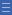## Featured resource

Home > Topdrawer > Mental computation > Activities > Equal differences

# Equal differences

Equal differences

Difference problems can be made easier by adjusting both numbers by the same amount.

Many pairs of numbers can share the same difference. The difference is easier to work out with some pairs than with others. Use small numbers at first.

Record the results symbolically so students can identify patterns. Generalise the idea that adding or subtracting the same number from both numbers in any pair conserves the difference between them.

Progress to problems in which finding differences between two-digit numbers is made easier by adding or subtracting the same amount from both numbers.

For example, 46 and 93 have the same difference as 50 and 97 (4 has been added to both numbers).

You can watch the Equal Additions video.

Students can practise finding pairs of numbers that share the same difference using the digital learning object L110 The difference bar: make your own hard subtractions.

These concepts are further explained in the big ideas.

Yes

Yes

Name Class SectionYear 4: Apply place value to partition, rearrange and regroup numbers to at least tens of thousands to assist calculations and solve problems Infobox 3Year 3: Recognise and explain the connection between addition and subtraction Infobox 3Year 3: Apply place value to partition, rearrange and regroup numbers to at least 10 000 to assist calculations and solve problems Infobox 3Source Infobox 3